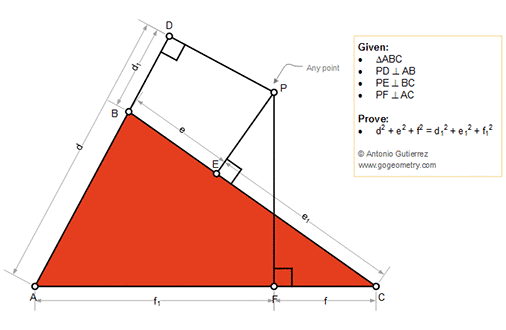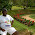## Friday, September 28, 2018

### Geometry Problem 1390: Triangle, any point, perpendicular, sum of the squares, Tutoring

Geometry Problem. Post your solution in the comment box below.
Level: Mathematics Education, High School, Honors Geometry, College.

Details: Click on the figure below.#### 3 comments:

1.connect PA,PB,PC;
e1^2-f^2=PF^2 - PE^2;
d1^2-e^2=PE^2-PD^2;
f1^2-d^2=PD^2-PF^2;
add together, right side =0;
so e1^2+d1^2+f1^2=e^2+d^2+f^2;

2.Let PD = x, PE = y & PF = z

Using Pythagoras 6 times

PA^2 = d^2 + x^2 = f1^2 + z^2 ....(1)
PB^2 = e^2 + y^2 = d1^2 + x^2 ...(2)
PC^2 = f^2 + z^2. = e1^2 + y^2 ...(3)

Adding these 3 equations together the result follows

Sumith Peiris
Moratuwa
Sri Lanka

3.Connect PA, PB, PC
Apply Pythagoras theorem we have
e^2-e1^2= PB^2-PC^2
f^2-f1^2= PC^2-PA^2
d^2-d1^2= PA^2-PB^2
add these expressions side by side we have d^2+e^2+f^2= d1^2+e1^2+f1^2